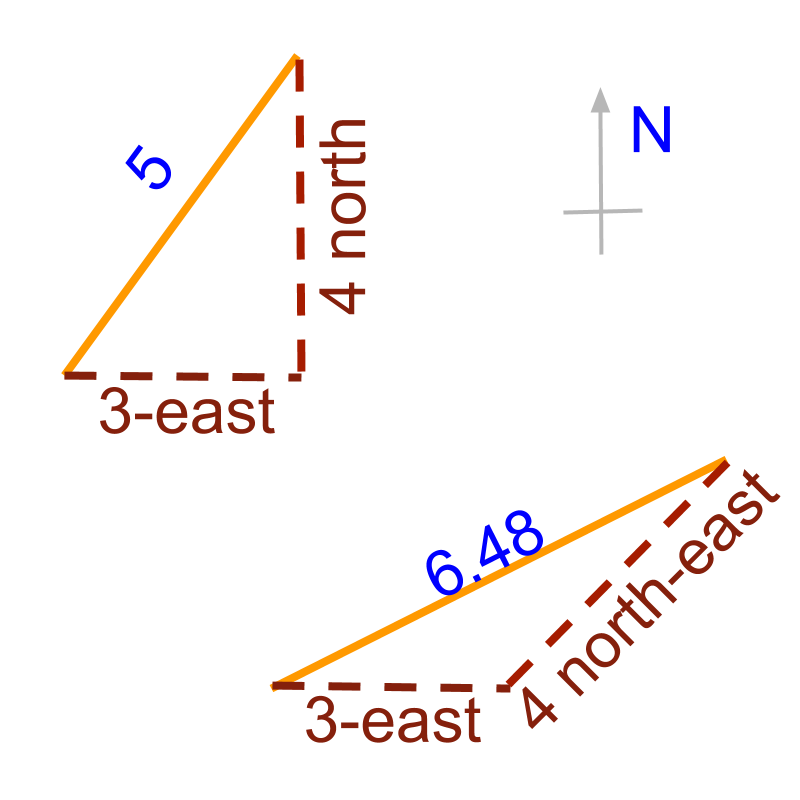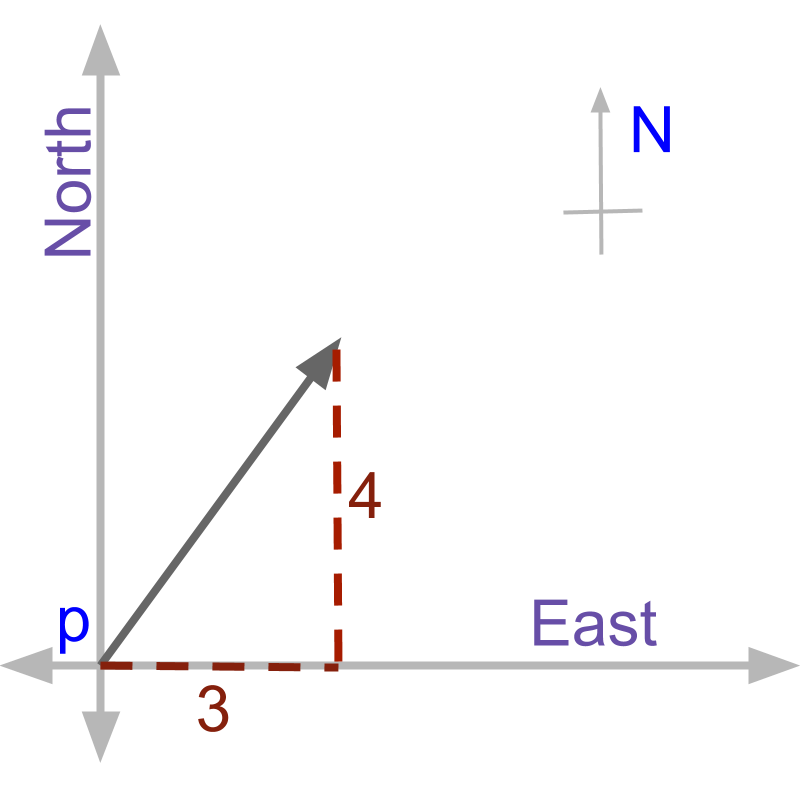maths > vector-algebra

Introducing Vectors

what you'll learn...

Overview

»  Measurement of Quantities
→  Addition: sum of measured amount
→  Subtraction: difference in measured amount

»  Some quantities do not add or subtract only in the amount→  $3$$3$ east + $4$$4$ north is not $7$$7$
→  $3$$3$ east + $4$$4$ north-east is approximately $6.48$$6.48$

»  Some quantities cannot be used in calculation if only amount is given without direction

»  Measurement of such quantities should capture both the amount and the direction to carryout calculations

set-up

One of the fundamental aspects of science is to measure and specify quantities. Examples:

•  mass of an object: $20$$20$ gram

•  temperature of water: ${30}^{\circ }$${30}^{\circ}$ Celsius

•  the amount of electric current: $5$$5$ ampere

•  the amount of time taken: $3$$3$ seconds

•  the amount of distance traveled: $20$$20$ meter

•  $20$$20$ gram mass and $12$$12$ gram mass together weigh $32$$32$ gram

•  ${30}^{\circ }$${30}^{\circ}$ Celsius heated up to add another ${7}^{\circ }$${7}^{\circ}$ Celsius make ${37}^{\circ }$${37}^{\circ}$ Celsius

•  $5$$5$ ampere current with another $1.2$$1.2$ ampere current added makes $6.2$$6.2$ ampere

•  $3$$3$ second walk and another $5$$5$ second walk make total $8$$8$ second walk

•  $20$$20$ meter distance walked and another $48$$48$ meter distance make $68$$68$ meter walked in total

Subtraction of quantities

•  $3$$3$gm mass is taken away from $20$$20$gram -- results in $17$$17$ gram

•  ${30}^{\circ }$${30}^{\circ}$ Celsius cooled down by ${38}^{\circ }$${38}^{\circ}$ -- results in $-{8}^{\circ }$$- {8}^{\circ}$ Celsius

•  $5$$5$ ampere current source has a $20$$20$ ampere current flowing in opposite direction will make $-15$$- 15$ ampere

•  planning a $3$$3$ second walk and then reducing the time by $1.2$$1.2$ second result in $1.8$$1.8$ second walk

•  planned $20$$20$ meter distance walk but stopped $5$$5$ meters before the end point, this results in $15$$15$ meter distance

With this basic of

•  measuring quantities,

•  addition and subtraction of them

We have a question.

•  Is amount of a quantity sufficient to completely specify quantities?

To understand the question, Let us look at another problem.

illustrative example

A person walks in a football ground. At first he walked $4$$4$ meters and then another $3$$3$ meters. How far he is from the starting point?

One student says, $4 + 3 = 7$

Another says $4 - 3 = 1$

Another calculates as $\sqrt{{4}^{2}+{3}^{2}}$$\sqrt{{4}^{2} + {3}^{2}}$

Any one of them can be correct.

It is not possible to find the distance in that question. Because the direction is not given. That is, whether the person continues in the same direction or the person changes direction in between is not given.

If the person walks $4$$4$ meters straight and continues in the same direction for another $3$$3$ meters, then $4 + 3 = 7$, as the distances are added when the directions are same.

If the person walks $4$$4$ meters straight. He turns around towards the starting point and walks $3$$3$ meters, Then $4 - 3 = 1$, as the person walked back $3$$3$ meter in the opposite direction.

Let us take a person walking from position 'p', $3$$3$ meter towards east and another $4$$4$ meter towards north. Then the straight distance between the end position and the start position is 'NOT $3 + 4$' and 'NOT $3 - 4$' either.The problem is illustrated    in figure. A line of $3$$3$ meter and another line of $4$$4$ meter perpendicular to the first line are shown. We can solve this using Pythagoras Theorem, which helps in finding hypotenuse of a right angle triangle using the two sides.
$3$$3$ meter and $4$$4$ meter are two sides of a right angled triangle and so
$\text{distance}=\sqrt{{3}^{2}+{4}^{2}}$$\textrm{\mathrm{di} s \tan c e} = \sqrt{{3}^{2} + {4}^{2}}$

direction

Apart from the 'amount' of distances the person is walking, it is important to note down the 'direction' in which the person is walking.

Measurement of some quantities require not just the amount , also the direction.

To completely specify and mathematically use, some quantities require both magnitude (amount) and direction. Such quantities are named as Vectors

magnitude: The measure of amount of a quantity.

Direction: The measure of relative position or orientation of a quantity.

understanding direction

Identify the statement in which direction information is specified.
He walks on grass.
He walks along with his friend.
He walks towards east.
He walks towards his success.

In the above, 'he walks towards east' specifies direction 'east'.

Identify the statement in which direction information is specified.
She felt the gravity pulling down.
She understood the gravity of the situation.
Gravitational force in Earth is higher than that of Moon

In the above, 'She felt the gravity pulling down' specifies direction 'downwards'.

Identify the statement in which direction information is specified.
Friction makes it possible to walk.
Friction acts against the movement.
friction causes wear.

In the above, 'friction acts against the movement' specifies direction 'against the movement'.

Identify the statement in which direction is specified.
On hearing the news, he smiled.
He spilled coffee.
He throws the ball towards me.

In the above, 'He throws the ball towards me' specifies direction 'towards' a reference.

Identify the statement in which direction is specified.
Wind blew at ${45}^{\circ }$${45}^{\circ}$angle.
Wind uprooted a tree weighing 250kg.
Wind caused the fan to rotate fast.

In the above, 'wind blew at ${45}^{\circ }$${45}^{\circ}$angle' specifies direction 'at ${45}^{\circ }$${45}^{\circ}$angle'.

number system

In "number systems", we have learned the following.

Whole numbers are $0,1,2,\cdots$$0 , 1 , 2 , \cdots$. These are used to count or measure quantities.

Whole numbers representation is not sufficient to represent directed numbers.

For example, consider the numbers in
• I received $3$$3$ candies and
& bull; I gave $3$$3$ candies.

In the whole numbers, both these are represented as $3$$3$.

In integers, the first is $+3$$+ 3$ and the second is $-3$$- 3$.

Integer numbers are represented as follows.

$3$$3$ is represented as either $\text{received:}3$$\textrm{\left(r e c e i v e d\right\rangle} 3$ or \text{aligned:}3$\textrm{\left(a l i g \ne d\right\rangle} 3$.

$-3$$- 3$ is represented as either $\text{given:}3$$\textrm{\left(g i v e n\right\rangle} 3$ or $\text{opposed:}3$$\textrm{\left(o p p o s e d\right\rangle} 3$.

Integers are directed whole numbers.

Whole numbers and Integers representation is not sufficient to represent quantities of part of an object.

For example, A pizza is cut into $8$$8$ pieces.

$3$$3$ whole pizzas and $5$$5$ pieces of a cut pizza are remaining.

Whole numbers or integers represent them as two quantities: $3$$3$ pizzas and $5$$5$ pieces when one whole is cut into $8$$8$ pieces. This representation is descriptive.

The same in fractions is $3\frac{5}{8}$$3 \frac{5}{8}$.

The same in decimals is $3.625$$3.625$

Fractions are numbers representing part of a whole. A whole is split into $q$$q$ number of equal sized pieces. The quantity specified is count $p$$p$ of the said equal sized pieces. This is given as fraction $\frac{p}{q}$$\frac{p}{q}$, where $p$$p$ is the count and $\frac{1}{q}$$\frac{1}{q}$ is the place value of the count.

Decimals are fractions in a standard form. Fractions are specified with different place values $\frac{1}{q}$$\frac{1}{q}$. Decimals are specified with standard place values $\frac{1}{10}$$\frac{1}{10}$, $\frac{1}{100}$$\frac{1}{100}$, $\frac{1}{1000}$$\frac{1}{1000}$, etc.

spatial direction

It is noted that the direction specified in the integers refers to

•  positive OR \text{aligned:}$\textrm{\left(a l i g \ne d\right\rangle}$

•  negative OR $\text{opposed:}$$\textrm{\left(o p p o s e d\right\rangle}$

The Integers are directed-whole-numbers, having positive integers and negative integers.

The fractions are also in directed form, having positive fractions and negative fractions. Likewise for decimals, positive decimals and negative decimals.

The direction information of vectors refers to the spatial direction,

•  x-axis (eg: right and left for a person)

•  y-axis (eg: forward and backward for a person) and

•  z-axis (eg: up and down for a person)

Note, the right-left, forward-backward, up-down are one particular example. It can also be east-west, north-south, up-down. Or it can be in other forms.

The values of a vector along the given spatial directions can be positive or negative (meaning aligned or opposed in direction).

Hope you understand the difference between

•  direction specified for positive and negative numbers. And

•  the spatial direction specified for vectors in 2-dimensions or 3-dimensions

A Vector: A quantity that requires both magnitude and direction information to process mathematically.

The word 'vector' means 'to direct towards a point'. Like in the sentence, 'the aeroplane is vectored towards New York.'

without direction

There are other quantities that are measured with only 'magnitude' and do not have 'direction' information.

Examples:
•  Mass of an object
•  temperature of water
•  the amount of time taken

The quantities which are specified only by 'magnitude' or 'amount' are called as Scalars

Scalar: Quantity that requires only magnitude information to process mathematically.

The meaning of the word 'scalar' is derived from 'to scale ; to measure'. It is used in mathematics to denote quantities with only magnitude.

A scalar quantity does not have direction as part of the measurement.

When measured, a vector quantity is specified by both magnitude and direction.

examples

A person, facing east, measures the temperature of the glass of water as ${25}^{\circ }$${25}^{\circ}$C. Is this measure scalar or vector?
The answer "a scalar". The person facing East does not add to the measure, rather an irrelevant information.

A person, measures the distances between three points on the floor as AB$= 20$cm, BC$= 10$cm, and AC$= 15$cm. Are these measurements scalars or vectors?

The points on the floor forms a triangle and direction information can be derived as angle between line segments. So, these are implicitly vectors with only magnitudes of them provided.

A person, measures the distances between three points on the floor as AB$= 20$cm and BC$= 10$cm. Are these distances scalars or vectors?

These are supposed to be vector quantities. The direction information is not measured. Thus the measurement is incomplete.

summary

Scalar: Quantity that requires only magnitude information to process mathematically.

A Vector: A quantity that requires both magnitude and direction information to process mathematically.

Outline